# 一、递归

• 这个问题可以转化为一个或多个子问题来求解，而且这些子问题的求解方法与原问题完全相同
• 递归调用的次数是有限的
• 必须有终止条件

• 对问题$f(s_{n})$进行分析，假设出合理的小问题$f(s_{n-1})$
• 假设小问题$f(s_{n-1})$是可解的，在此基础上确定大问题$f(s_{n})$的解，即给出$f(s_{n})$与$f(s_{n-1})$之间的关系
• 确定一个特殊情况（如$f(s_{1})$或$f(s_{0})$的解），由此作为递归出口

def recursion(level, param1, param2, ...):
# 递归终止条件
if level > MAX_LEVEL:
return result
# 当前level的逻辑
process_data(level, data)
# 递归
self.recurison(level, param1, param2, ...)
# 如果必要的话，还原此层的状态
reverse_state(level)

# 二、例题

## 1、两两交换链表中的节点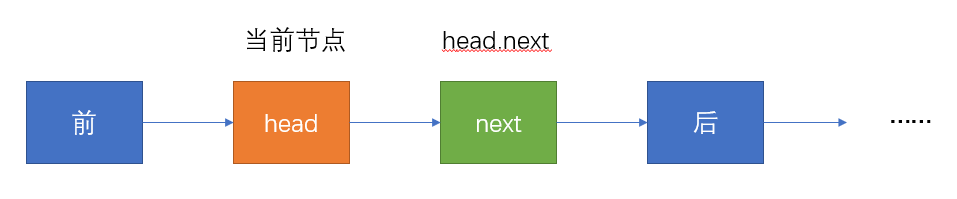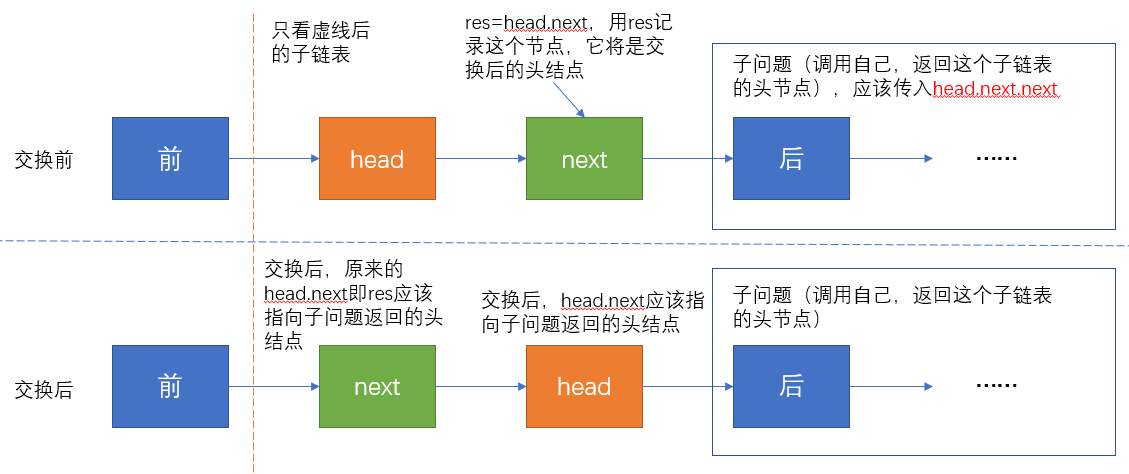• 我们希望headnext互换，互换后head指向后面，next变为当前子链表的头结点
• 交换后，head应该指向后面的子链表，而后面的子链表应该是交换完成了的，也就是说，后面的子链表两两交换是个子问题，解决方式和当前的方式一样，所以这个子链表的头结点（即head.next.nex应该传入递归函数中）
• 因为交换后next为当前子链表的头结点，我们用一个指针指向它，即res=head.next，交换完后我们返回res即可，因为当前子链表也是上一层递归调用的子问题，我们要返回当前链表的头结点
• 交换的过程：首先我们需要将head.next指向后面子问题返回的头结点，然后next指向head，即res.next=head
• 交换完后，返回此时当前子链表的头结点res即可
• 终止条件：当head=None（无节点）或head.next=None（此时为最后一个节点）时，直接返回head
class Solution:
def swapPairs(self, head: ListNode) -> ListNode:
# 终止条件
# 交换后的头结点
return res
• 时间复杂度：$O(N)$，其中 $N$ 指的是链表的节点数量
• 空间复杂度：$O(N)$，递归过程中使用的堆栈空间

## 2、反转链表

此题为leetcode第206题
示例：输入: 1->2->3->4->5->NULL 输出: 5->4->3->2->1->NULL
假设我们有如下链表：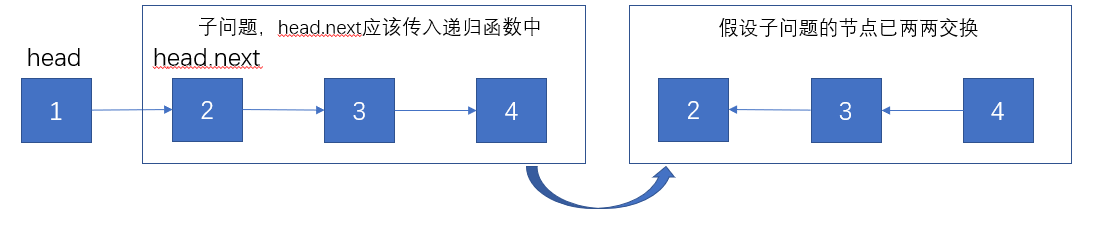当前节点为head，假设子问题返回的链表已经两两反转，我们希望节点2能指向节点1，节点1指向None。递归过程如下图所示：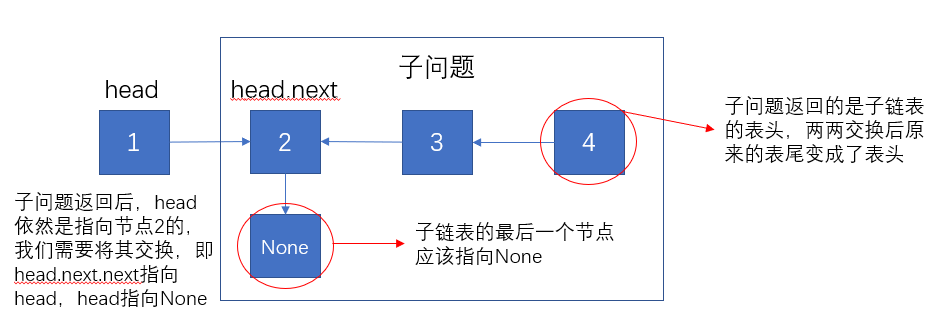• 当前节点为head，我们期望后续的子链表指向head，而head指向None。那么后续子链表的两两交换问题为子问题。

• 子问题里的子链表节点两两交换后，原来的尾节点（图中的节点4）变成了头结点，并且要返回这个头结点；原来的头结点（图中的节点2）变为了尾节点，并且要指向None，因为尾节点最后都要指向None。注意此时head节点依然是指向节点2的，因为子问题并没有改变head的指向
• 交换过程：需要将节点2指向节点1，即head.next.next=head，然后head指向None
• 终止条件：head=None（无节点）或head.next=None（最后一个节点）时，直接返回head
class Solution:
def reverseList(self, head: ListNode) -> ListNode:
# 终止条件
# 子问题递归，返回交换完后的子链表的头结点
# 交换
return res
• 时间复杂度：$O(N)$，其中 $N$ 指的是链表的节点数量
• 空间复杂度：$O(N)$，递归过程中使用的堆栈空间

## 3、斐波那契数列

记忆化
此题为leetcode第509题。斐波那契数列有如下递归关系：给定$n$计算$f(n)$。以计算$f(4)$为例，我们可以得到：$f(4)=f(3)+f(2)=f(2)+f(1)+f(1)+f(0)=f(1)+f(0)+f(1)+f(1)+f(0)$，把这个递归过程画出来如下图所示：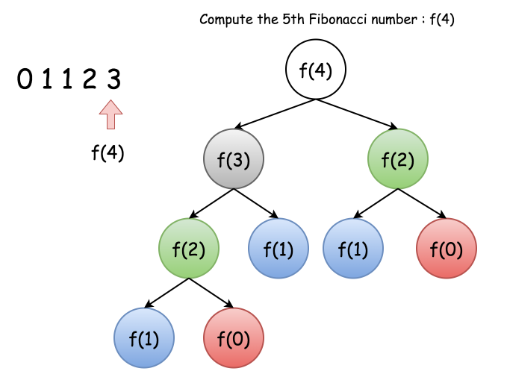按说这个很容易找到子问题，并且终止条件也明确，但是我们观察发现，这其中有很多重复的计算，比如$f(2)$重复了两次，这会导致内存占用较多。为此，我们可以将中间结果暂存起来，到时候直接用就可以。
class Solution:
def fib(self, N: int) -> int:
# 缓存字典
catch = {}
def rec(N):
# 若N在缓存中，直接返回对应值
if N in catch:
return catch[N]
# N小于2时直接返回自己
if N < 2:
res = N
else:
res = rec(N-1) + rec(N-2)
# 放入缓存中
catch[N] = res
return res
return rec(N)
• 时间复杂度：$O(N)$
• 空间复杂度：$O(N)$，缓存空间大小

## 4、合并两个有序链表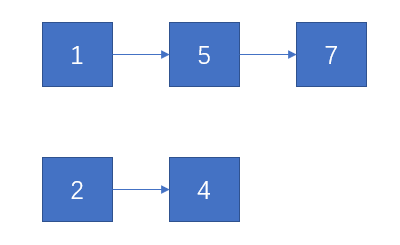l1指向了第一个链表中的某个位置，l2指向了第二个链表中的某个位置，我们比较这两个节点的大小。如果l1<l2，那么l1应该在l2之后，l2应该和l1之后的子链表继续比较，由此形成子问题。如果l1>l2，那么l2应该在l1之后，l1继续和l2之后的子链表比较。整个过程如下所示：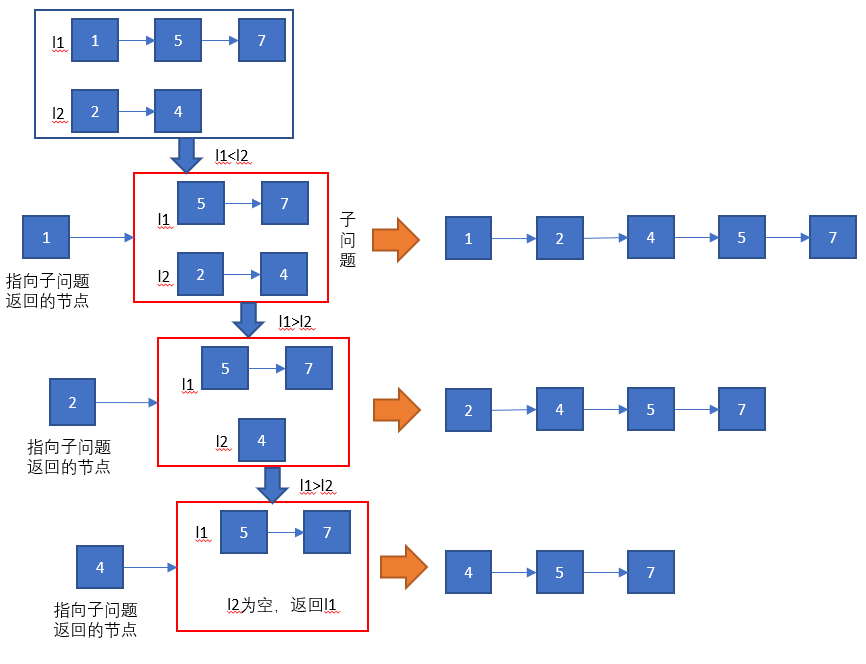• l1<l2，则将l1.nextl2传入递归函数中，将l1指向递归函数返回的节点；反之将l2.nextl1传入递归函数中，l2指向递归函数返回的节点。
• l1=None时，说明l1已提前遍历完（或l1本来为空），l2剩下的也不用比较了，直接返回l2；同理l2=None时，直接返回l1
class Solution:
def mergeTwoLists(self, l1, l2):
if l1 is None:
return l2
elif l2 is None:
return l1
elif l1.val < l2.val:
l1.next = self.mergeTwoLists(l1.next, l2)
return l1
else:
l2.next = self.mergeTwoLists(l1, l2.next)
return l2
• 时间复杂度：$O(n + m)$。因为每次递归调用都会将指向 l1l2 的指针递增一次（逐渐接近每个列表末尾的 null），所以每个列表中的每个元素都会对 mergeTwoLists 进行一次调用。 因此，时间复杂度与两个列表的大小之和是线性相关的。
• 空间复杂度：$O(n + m)$。一旦调用 mergetwolist，直到到达 l1l2 的末尾时才会返回，因此 $n + m$的栈将会消耗 $O(n + m)$ 的空间。

## 5、Pow(x, n)

class Solution:
def myPow(self, x: float, n: int) -> float:
# 递归1
# def func(x, n):
#     if n == 0:
#         return 1
#     if x == 0:
#         return 0
#     temp = func(x, n >> 1)
#     # 位运算判断奇偶
#     if n & 1: # n为奇数
#         return temp * temp * x
#     else: # n为偶数
#         return temp * temp
# if n >= 0:
#     res = func(x, n)
# else:
#     res = 1 / func(x, -n)
# return res

# 递归2
# if n == 0:
#     return 1
# if n < 0:
#     return 1. / self.myPow(x, -n)
# if n % 2:   # 如果n是奇数，在下一层算n/2
#     return x * self.myPow(x, n - 1)
# else:       # 如果n是偶数
#     return self.myPow(x * x, n/2)

# 非递归
if n < 0:
x = 1. / x
n = -n
res = 1
while n:
if n & 1:   # n为奇数
res *= x
x *= x
n >>= 1 # 右移一位，相当于n // 2
return res